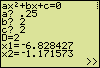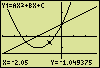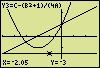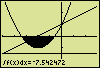# Parabolaa program for the Texas Instruments TI-83and TI-84 (Plus) graphing calculators

### Summary

The equation y=ax²+bx+c is a mathematical representation of all parabolas with the axis of symmetry parallel to the y-axis. To select a particular parabola from the set as a whole, the parameters a, b and c need to get the matching numerical values. So, if the program Parabola has to examine an individual case, it will ask the user to put in the three numbers.

At first the quadratic equation ax²+bx+c=0 is evaluated. Using the values of a, b and c the program calculates the discriminant D and the real roots x1 and x2 (as far as they exist).By request a diagram is shown. The following lines are displayed:
- the parabola y = ax2+bx+c  (parabola Y1 at the screen);
- the derivative y' = dy/dx = 2ax+b  (oblique line Y2);
- the directrix y = c–(b2+1)/(4a)  (horizontal line Y3);
- and both axes (y=0 and x=0).From now all calculator functions are available. Move the cursor along a line (left/right arrow), jump to another line (up/down arrow), scroll the diagram by moving the cursor position to the centre of the screen (ENTER) or put in an x-value and read off the new cursor coordinates at the bottom line. Perform calculations leading to for instance the coordinates of the parabola top or a point of intersection, or determine an area as done below (CALC, TABLE).The coordinate axes
The program pursues to compose diagrams in which the x- and y-axis have identical divisions. In several cases however, if the underlying procedure tends to generate a scarcely recognizable parabolic curve, the program takes action to produce a more satisfying picture. After such an intervention the divisions of the axes aren't identical any more and the message "Axes differ" is displayed instead of "Axes equal". Press WINDOW to see the actual settings.

Using D, x1, x2
To keep any imaginable result, such as x2=-1.234567E-12, within one line at the screen, the roots to show, x1 and x2, are rounded to 7 'significant' figures. The discriminant holds at most 8 of them (in the example above the noughts are omitted).
Even though hidden from view, the remaining decimals are still available: end the program and recover the unprocessed results from the memories D, E and F at the basic screen. In case of the given example, pressing ALPHA, E, ENTER reveals an x1 of -6,828427125 (-6,8284271247462).At the graph screen you can retrieve these data to have a question answered. If you type for instance (E+F)/2 followed by ENTER, the parabola top is marked and the coordinates of the top are displayed at the bottom line.
In particular when working with an item selected from the CALC menu, the option may be very useful. The already mentioned area determination is a good example.
Finally an important note: if D<0 (no real roots) the values stored in E and F (1) are meaningless.

D = b2–4ac
x1 = (-b–D0,5)/(2a)
x2 = (-b+D0,5)/(2a)
xtop = -b/(2a)
ytop = -D/(4a)
directrix: y = -(D+1)/(4a)
distance from top to directrix = abs(1/(4a))

Errors and peculiarities
• If the displayed directrix is a dotted line...
Your screen's resolution setting is greater than one (Xres>1). Press WINDOW to see the actual Xres value.
• If not all expected lines (Y1,Y2,Y3) are displayed...
Press the "Y=" key to view the equations Y1, Y2 and Y3, all of which should be highlighted. If not so, and in addition the WINDOW screen shows an option "SETTINGS", uninstalling the application "Transfrm" may solve the problem. Start up the application. If a menu appears, select "1:Uninstall", press ENTER (this will not delete the application) and restart the program "PARABOLA". If the initial screen does not offer an uninstall option, press 2nd, OFF (any other keystroke will install "Transfrm").

### Program Listing

[ Remarks ]
PROGRAM:PARABOLA
"Mem L3,L4,Y1-Y4,A-H,Y,Z"
"ver200411"
Normal:Float
Full
ClrHome
Disp "** Parabola **","ax2+bx+c=0","parameters a,b,c"
Disp "Output:","D, roots x1,x2","Draw graph of"
Output(8,1,"(1:yes,0:no)")
Input "y=ax2+bx+c ? ",G
Lbl 1
ClrHome
Disp "ax2+bx+c=0"
Output(3,1,"(0:stop)")
Input "a? ",Z
If Z=0
Then
ClrHome
Stop
End
Z→A
Input "b? ",B
Input "c? ",C
B^2–4AC→D
.5/A→H
Disp "D="
10^int(log(max(10^-90,abs(D)))–7)→Z
[ negation symbol (-) in "-90" ]
[ subtraction symbol in "–7" ]
Output(5,3,Zround(D/Z,0))
If D<0
Then
Disp "Discriminant<0","No real roots"
1→E:1→F
Else
(-B–D^.5)H→E
(-B+D^.5)H→F
Disp "x1=","x2="
10^int(log(max(10^-90,abs(E)))–6)→Z
Output(6,4,Zround(E/Z,0))
10^int(log(max(10^-90,abs(F)))–6)→Z
Output(7,4,Zround(F/Z,0))
End
Output(8,15,">>")
Pause
If G≠1
Goto 1
Sequential
Func:RectGC
CoordOn:GridOff
AxesOn:LabelOff
ExprOn:PlotsOff
"AX3/3+BX2/2+CX"→Y4
FnOff
GraphStyle(1,1)
GraphStyle(2,1)
GraphStyle(3,7)
GraphStyle(4,1)
"AX2+BX+C"→Y1
"2AX+B"→Y2
"C–(B2+1)/(4A)"→Y3
4→dim(L3)
4→dim(L4)
min(0,-BH)→L3(1)
max(0,-BH)→L3(2)
2abs(H)→Z
If B=0
Then
-Z→L3(1)
Z→L3(2)
End
-.5DH→Y
Y+3H→Z
If D<0
2Y→Z
Y–.5H→G
min(min(0,G),min(Y,Z))→L3(3)
max(max(0,G),max(Y,Z))→L3(4)
L3(2)+L3(1)→L4(1)
1.5(L3(2)–L3(1))→L4(2)
L3(4)+L3(3)→L4(3)
1.5(L3(4)–L3(3))→L4(4)
L4/2→L4
L4(4)/L4(2)*94/62→Z
If Z<1
L4(4)/Z→L4(4)
If Z>1
L4(2)*Z→L4(2)
If A>0
Then
L4(3)+L4(4)→Z
Else
L4(3)–L4(4)→Z
End
(B^2–4A(C–Z))^.5/abs(A)→Z
2L4(2)/Z→Z
0→G
If Z>5
Then
1→G
1.5L4(2)/Z→L4(2)
End
L4(1)–L4(2)→L3(1)
L4(1)+L4(2)→L3(2)
L4(3)–L4(4)→L3(3)
L4(3)+L4(4)→L3(4)
10^int(log(max(10^-90,abs(L3)))–1)→L4
int(L3/L4)*L4→L3
L3(1)→Xmin
L3(2)→Xmax
L3(3)→Ymin
L3(4)→Ymax
max(10^-90,(Ymax–Ymin)/5)→Y
10^int(log(Y))→Z
int(Y/Z)→Y
If Y>5
5→Y
If Y≠5
min(Y,2)→Y
ZY→Xscl
Xscl→Yscl
ClrHome
Disp "Lines to draw:","- y=ax2+bx+c","- dy/dx=2ax+b=y'"
Disp "- directrix","- axes (y=0,x=0)","","info:"
Output(8,1,"www.tenhorn.com")
Output(7,15,">>")
Pause
ClrHome
If G=1
Then
Disp "(Axes differ)"
Else
Disp "(Axes equal)"
End
Disp "","Press TRACE,","left/right arrow","","(adjust WINDOW","or ZOOM)"
Output(8,1,"")
Stop

If you need help with programming your calculator, this exercise will put you on the way: Creating a Program for the TI-83 calculator

Hein ten Horn
Top | Programs Texas Instruments | Home